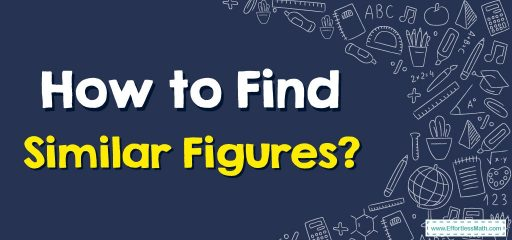# How to Find Similar Figures?

Similar figures mean that two shapes are the same shape but have different sizes. In this guide, you will learn more about finding similar figures.Two shapes are called similar when both have the same properties but may not be the same. For example, the sun and the moon may seem the same size, but in reality, they are different sizes.

## A step-by-step guide tofinding similar figures

In geometry, when two shapes like triangles, polygons, quadrilaterals, etc. have common dimensions or proportions but the size or length is different, they are considered similar figures. For example, two circles (of any radius) have the same shape but different sizes because they are similar. Look at the picture below.

The symbol for expressing similar figures is the same symbol for congruence i.e. “$$∼$$” but similarly does not mean the same in size. When the ratios of the corresponding sides are equal, the shapes are considered similar, that is, when dividing each set of corresponding side lengths, the number obtained is the scale factor. This number helps increase or decrease the size of the figures, but not in shape leaving them looking like similar figures.

### Similarity of triangles

Two triangles will be similar if the angles are equal (corresponding angles) and the sides are in equal proportion (corresponding sides). Similar triangles may have different lengths of sides of triangles, but their angles must be equal and their corresponding ratio of the lengths of the sides, or the scale factor must be the same.

If two triangles are similar that means,

• All corresponding angle pairs of triangles are equal.
• All corresponding sides of triangles are proportional.

### Similarity of polygons

Similar polygons have the same shape but differ in size. There will be certain uniform ratios in similar polygons. In other words, the corresponding angles are congruent, but the corresponding sides are proportional.

There are two important properties of similar polygons:

• The corresponding angles are equal/congruent. (Both interior and exterior angles are the same)
• The ratio of the corresponding sides is the same for all sides. Hence, the perimeters are different.

Quadrilaterals are polygons that have four sides. The sum of the interior angles of a quadrilateral is $$360$$ degrees. Two quadrilaterals are similar when the three corresponding angles are the same and the two adjacent sides have equal ratios.

## Exercises for Finding Similar Figures

### Each pair of figures is similar. Find the missing side.

1. $$\color{blue}{100}$$
2. $$\color{blue}{9}$$
3. $$\color{blue}{12}$$

### What people say about "How to Find Similar Figures? - Effortless Math: We Help Students Learn to LOVE Mathematics"?

No one replied yet.

X
30% OFF

Limited time only!

Save Over 30%

SAVE $5 It was$16.99 now it is \$11.99